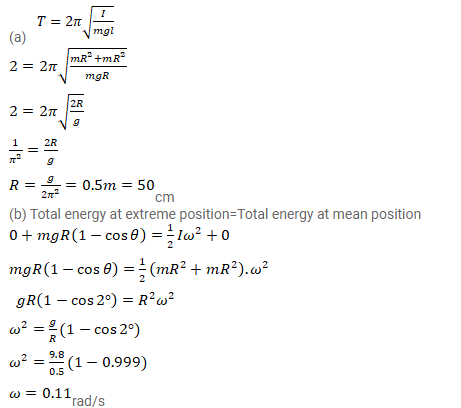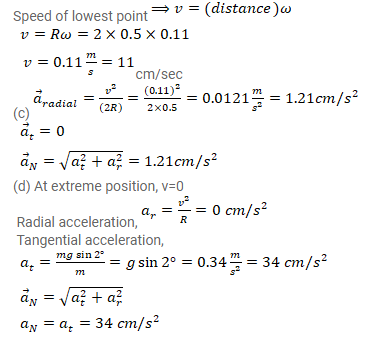# A closed circular wire hung on a nail in a wall undergoes small oscillations

Question:

A closed circular wire hung on a nail in a wall undergoes small oscillations of amplitude $2^{\circ}$ and time period 2 s. Find (a) the radius of the circular wire, (b) the speed of the particle farthest away from the point of suspension as it goes through its mean position, (c) the acceleration of this particle as it goes through its mean position and (d) the acceleration of this particle when it is at an extreme position. Take $g=\pi^{2} \mathrm{~m} / \mathrm{s}^{2}$.

Solution: